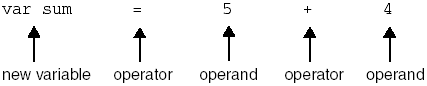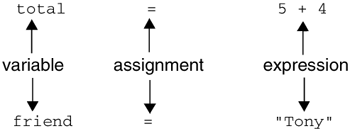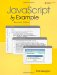# 5.1 About JavaScript Operators and Expressions

Data objects can be manipulated in a number of ways by the large number of operators provided by JavaScript. Operators are symbols, such as +, “, =, >, and <, that produce a result based on some rules. An operator manipulates data objects called operands; for example, 5 and 4 are operands in the expression 5 + 4 . Operators and operands are found in expressions. An expression combines a group of values to make a new value, n = 5 + 4 . And when you terminate an expression with a semicolon, you have a complete statement (e.g., n = 5 + 4; ).In the numeric expression , 5 + 4 “ 2 , three numbers are combined. The operators are the + and “ signs. The operands for the + sign are 5 and 4 . After that part of the expression is evaluated to 9 , the expression becomes 9 2 . After evaluating the complete expression, the result is 7 . Since the plus and minus operators each manipulate two operands, they are called a binary operators. If there is only one operand, the operator is called a unary operator. If there are three operands, it is called a ternary operator. We'll see examples of these operators later in the chapter.

The operands can be either strings, numbers, Booleans, or a combination of these. We have already used some of the operators: the concatenation operator to join two strings together, the typeof operator to determine what data type is being used, and the assignment operator used to assign a value to a variable. Now let's look at a plethora of additional JavaScript operators and see how they manipulate their operands.

#### 5.1.1 Assignment

An assignment statement evaluates the expression on the right-hand side of the equal sign and assigns the result to the variable on the left-hand side of the equal sign. The equal sign is the assignment operator.

` `
` var total = 5 + 4; var friend = "Tony";`

#### 5.1.2 Precedence and Associativity

When an expression contains a number of operators and operands, such as 5 * 4 + 3 / “2.2 , and the order of evaluation is ambiguous, then JavaScript must determine what to do. This is where the precedence and associative rules come in. They tell JavaScript how to evaluate such an expression. Precedence refers to the way in which the operator binds to its operand, such as, should addition be done before division or should assignment come before multplication? The precedence of one operator over another determines what operation is done first. As shown in Table 5.1, the operators are organized as a hierarchy, the operators of highest precedence at the top, similar to a social system where those with the most power (or money) are at the top. In the rules of precedence, the multiplication operator is of higher precedence than the addition operator, technically meaning the operator of higher precedence binds more tightly to its operands. The assignment operators are low in precedence and thus bind loosely to their operand. In the expression sum = 5 + 4 the equal sign is of low precedence, so the expression 5 + 4 is evaluated first and then the result is assigned to sum . Parentheses are of the highest precedence. An expression placed within parentheses is evaluated first; thus, in the expression 2 * (10 “ 4) , the expression within the parentheses is evaluated first and that result is multiplied by 2 . When parentheses are nested, the expression contained within the innermost set of parentheses is evaluated first.

Associativity refers to the order in which an operator evaluates its operands: left to right in no specified order, or right to left. When all of the operators in an expression are of equal precedence, normally the association is left to right; in the expression 5 + 4 + 3 , the evaluation is from left to right. In Example 5.1, how is the expression evaluated? Is addition, multiplication, or division done first? And in what order, right to left or left to right?

In Table 5.1 the operators on the same line are of equal precedence. The rows are in order of highest to lowest precedence.

##### Table 5.1. Precedence and associativity.

Operator

Description

Associativity

()

Parentheses

Left to right

++ “ “

Auto increment, decrement

Right to left

!

Logical NOT

Right to left

* / %

Multiply, divide, modulus

Left to right

+ “

Left to right

+

Concatenation

Left to right

< <=

Less than, less than equal to

Left to right

> >=

Greater than, greater than equal to

Left to right

= = !=

Equal to, not equal to

Left to right

= = = != =

Identical to (same type), not identical to

Left to right

&

Bitwise AND

Left to right

Bitwise OR

^

Bitwise XOR

~

Bitwise NOT

<<

Bitwise left shift

>>

Bitwise right shift

>>>

Bitwise zero-filled, right shift

&&

Logical AND

Left to right

Logical OR

Left to right

? :

Ternary, conditional

Right to left

= += “ = *= /= %= <<= >>=

Assignment

Right to left

,

(comma)

##### Example 5.1
` <html><head><title>First JavaScript Sample</title> 1   <script language = "JavaScript"> 2  var result = 5 + 4 * 12 / 4;  3       document.write("<font size='+1'>result = " +  result  ,"<br>"); 4   </script>     </head>     <body bgcolor="yellow" text="blue"></body>     </html> `

EXPLANATION

1. This is the starting JavaScript tag.

2. The order of associativity is from left to right. Multiplication and division are of a higher precedence than addition and subtraction, and addition and subtraction are of higher precedence than assignment. To illustrate this, we'll use parentheses to group the operands as they are grouped by JavaScript. In fact, if you want to force precedence, use the parentheses around the expression to group the operands in the way you want them evaluated. The following two examples produce the same result:

` `
` var result = 5 + 4 * 12 / 4; `

could be written

` `
` result = (5 + ( ( 4 * 12 ) / 4)); `
3. The expression is evaluated and the result is assigned to variable, result . The value of result is displayed on the browser.

4. This is the closing JavaScript tag.

##### Figure 5.1. Output from Example 5.1.##### Example 5.2
` <html>     <head>     <title>Precedence and Associativity</title>     </head>     <body>     <script language = "JavaScript"> 1   var x = 5 + 4  * 12 / 4; 2   document.writeln( "<h3>The result is " +  x  + "<br>"); 3   var x = ( 5 + 4 )  * ( 12 / 4 ); 4   document.writeln("The result is " +  x  );     </script>     </body>     </html> `

EXPLANATION

1. The variable, called x , is assigned the result of the expression.

` `
` var x = 5 + 4 * 12 / 4; `

results in

` `
` x = 5 + 48 / 4 `

results in

` `
` x = 5 + 12 `

results in

` `
` 17 `

Since multiplication and division are higher on the precedence table than addition, those expressions will be evaluated first, associating from left to right.

2. The result of the previous evaluation, the value of x , is sent to the browser.

3. The expressions enclosed in parentheses are evaluated first and then multiplied.

` `
` var x = ( 5 + 4 ) * ( 12 / 4 ); `

results in

` `
` x = 9 * 3 `

results in

` `
` 27 `
4. The result of the previous evaluation, the value of x , is sent to the browser. The output of the script is shown in Figure 5.2.

##### Figure 5.2. Output from Example 5.2.JavaScript by Example (2nd Edition)
ISBN: 0137054890
EAN: 2147483647
Year: 2003
Pages: 150
Authors: Ellie Quigley

Similar book on Amazon